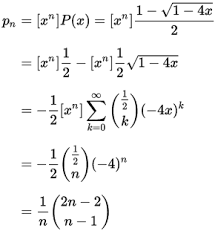# Enumerative Combinatorics: Home

Enumerative combinatorics. ... More generally, given an infinite collection of finite sets Si indexed by the natural numbers, enumerative combinatorics seeks to describe a counting function which counts the number of objects in Sn for each n.

## Study level

Postgraduate## Library Timing## What is Enumerative Combinatorics?

Enumerative combinatorics is an area of combinatorics that deals with the number of ways that certain patterns can be formed. Two examples of this type of problem are counting combinations and counting permutations. More generally, given an infinite collection of finite sets Si indexed by the natural numbers, enumerative combinatorics seeks to describe a counting function which counts the number of objects in Sn for each n. Although counting the number of elements in a set is a rather broad mathematical problem, many of the problems that arise in applications have a relatively simple combinatorial description.The twelvefold way provides a unified framework for counting permutationscombinations and partitions.The simplest such functions are closed formulas, which can be expressed as a composition of elementary functions such as factorials, powers, and so on. For instance, as shown below, the number of different possible orderings of a deck of n cards is f(n) = n!. The problem of finding a closed formula is known as algebraic enumeration, and frequently involves deriving a recurrence relation or generating function and using this to arrive at the desired closed form.## Information Executive